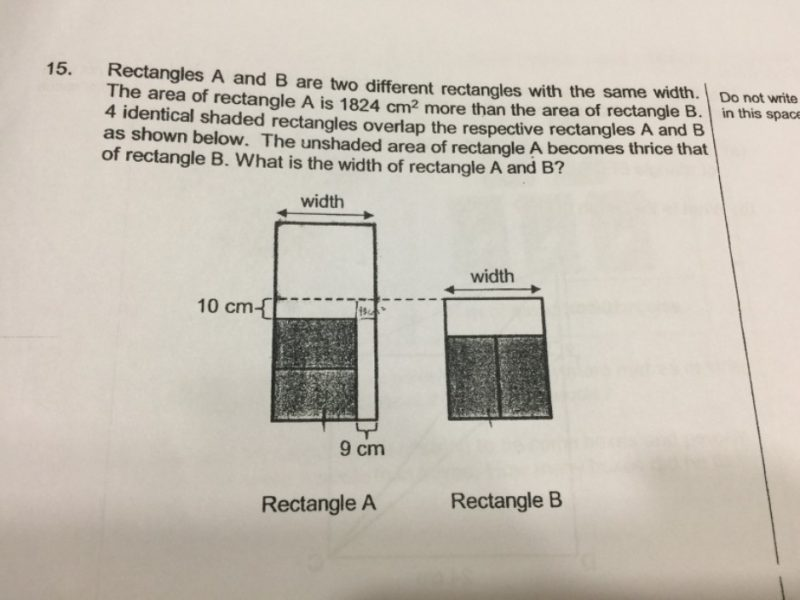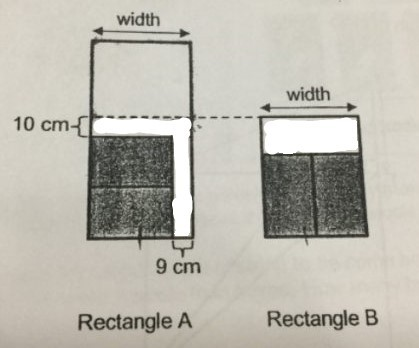# QuestionLet the breadth of the shaded rectangle be B, and the width be W.

Then the width W = 2B.

Unshaded area of rectangle A is 3 times the unshaded area of rectangle B.

1824 + 10W + 9(2B) = 3 (10W + 9(2B))

Since 2B = W,

1824 + 10W + 9W = 3 (10W + 9W)

38W = 1824

W = 1824 ÷ 38 = 48

The width is 48 cm.

0 Replies 0 Likes ✔Accepted AnswerThe area of the white part in Rectangle A is equal to the area of the white part in Rectangle B.

0 Replies 0 Likes